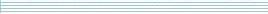| home      | people     | research     | publications    | seminars    | events     | contactCommunication Networks   | Systems Biology   | Hybrid Systems    | Machine Learning   | Dynamics & Interaction### Schools Mathematics Grand Challenge

Week five's Puzzles

Problem 13:

The solution was:

To start with, there are 500 cheaters and 49,500 noncheaters.

Let's count the people on the special list. There are 99/100 x 500 = 495 cheaters and 1/3/100 x 49,500 = 165 noncheaters on the list. So there is a total of 660 people on the list.

Now looking only at the special list, the percentage of cheaters is 495 / (660) = 75%.

This question demonstrates the dangers of statistics when you are testing for something rare. Here the test is very accurate; only .0033333 of the non cheaters get mislabelled!!! However because of the rarity of cheating, if the test says you are a cheat, there is a 25% chance it is wrong.

Many medical tests for diseases are good examples of this "problem".

Problem 14:

The solution was:

This problem like so many others is easier if you look at it the right way. Here we're going to look at it by imagining ourselves sitting on the back of of the hare, and only looking at the cheetah and the turtle, not at the scenery or ground.

What do we see from this perspective? We see the cheetah moving clockwise away from us at 13-7=6 laps per hour, and we see the hare moving anticlockwise away from us at 7-1=6 laps per hour. So they will both have done one relative lap and meet up with us again in 1/6 of an hour, which is ten minutes.

Problem 15:

The solution was:

This puzzle is an optical illusion. Although the two big triangles look like triangles, they are not. If they were triangles, they would have the same area - but this is impossible because of the gap and the fact that they are made up of the same pieces.

So to answer the question, we must use the smaller yellow and red triangles: Tan(A) =3/8 and Tan(B) = 2/5, and the answer is -3. (We also accepted 3 as a correct answer.)

The tangent represents the steepness of the hypotenuse - so the fact that the two hypotenuses have different steepness demonstrates that there is a corner where the yellow and red triangles meet, and that the big triangles only look like triangles.